## OpenCV中提取目标区域并标注

2021/2/7 11:09:27 文章标签: 测试文章如有侵权请发送至邮箱809451989@qq.com投诉后文章立即删除

### 提取目标区域并标注

• 前言
• 一、blob分析
• 二、有关代码函数的注释与用法
• 1、cv2.threshold（）阈值处理
• 2、cv2.findContours（）轮廓检测
• 3、cv2.boundingRect(c)矩形边框
• 4、cv2.moments()图像的矩
• 5、cv2.circle（）画圆心
• 6、 cv2.line（）画直线
• 7、cv2.putText（）写文字

# 前言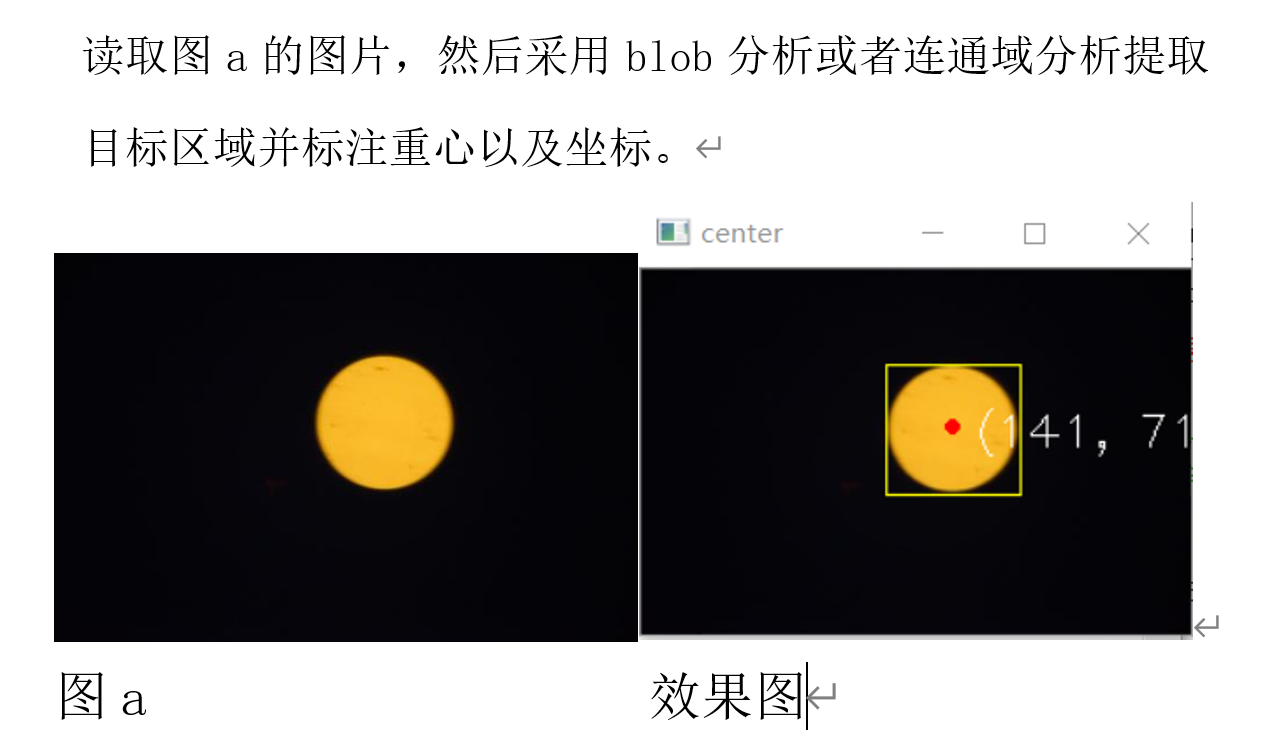# 一、blob分析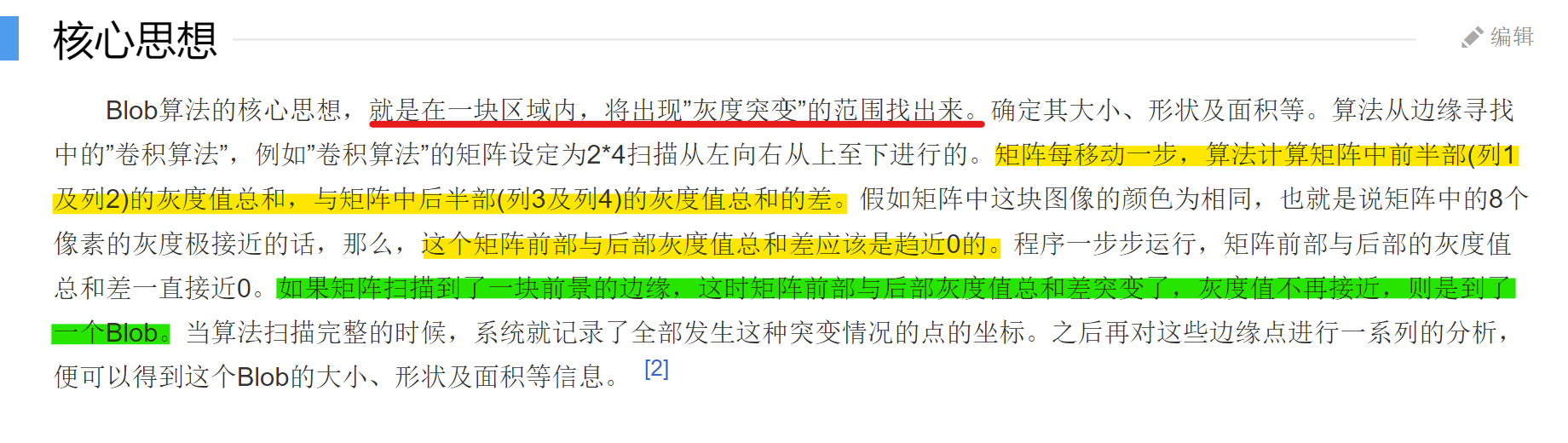``````import cv2
import numpy as np

imgray = cv2.cvtColor(img, cv2.COLOR_BGR2GRAY)#读取并处理为单通道图片，为后续做准备
ret, binary = cv2.threshold(imgray, 127, 255, 0)#阈值处理
contours, hierarchy = cv2.findContours(binary, cv2.RETR_TREE, cv2.CHAIN_APPROX_SIMPLE)#查找检测物体的轮廓
for c in contours:
(x, y, w, h) = cv2.boundingRect(c)
img = cv2.rectangle(img, (x, y), (x + w, y + h), (0, 255, 255), 1)
for i in range(len(contours)):
mom = cv2.moments(contours[i])#计算中心矩
pt = (int(mom['m10'] / mom['m00']), int(mom['m01'] / mom['m00']))  # 使用前三个矩m00, m01和m10计算重心
cv2.circle(img, pt, 2, (0, 0, 255), 2)  # 画红点
cv2.line(img, (x, y),((pt),(pt)), (0, 0, 255),1)#画半径
text = "(" + str(pt) + ", " + str(pt) + ")"#添加的文字
cv2.putText(img, text, (pt+10, pt+10), cv2.FONT_HERSHEY_PLAIN, 1.5, (255, 255, 255), 2, 8, 0);#加坐标

print("--------- HL分析区域并标注的测试 ---------")
cv2.imshow("center", img)
cv2.waitKey(0)
cv2.destroyAllWindows()
``````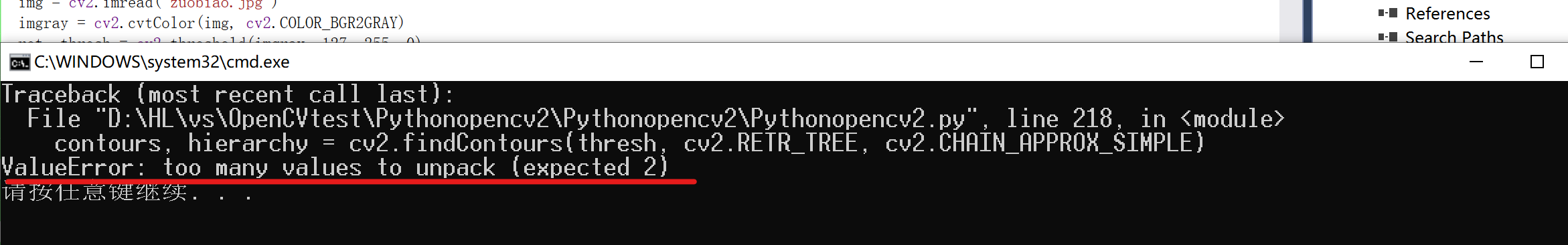OpenCV4.0的说明文档中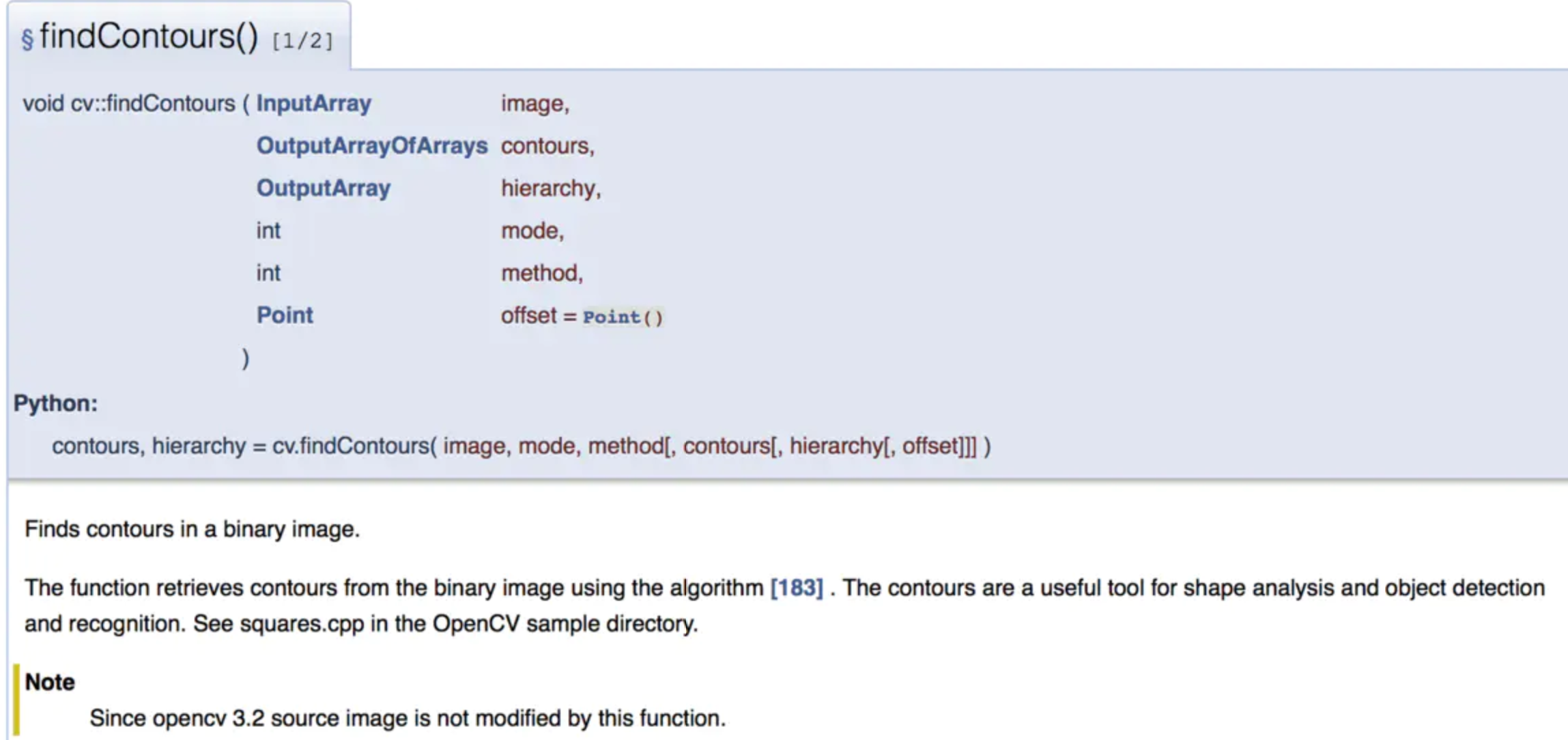``````contours, hierarchy = cv2.findContours(binary, cv2.RETR_TREE, cv2.CHAIN_APPROX_SIMPLE)
``````

``````image, contours, hierarchy = cv2.findContours(binary, cv2.RETR_TREE, cv2.CHAIN_APPROX_SIMPLE)
``````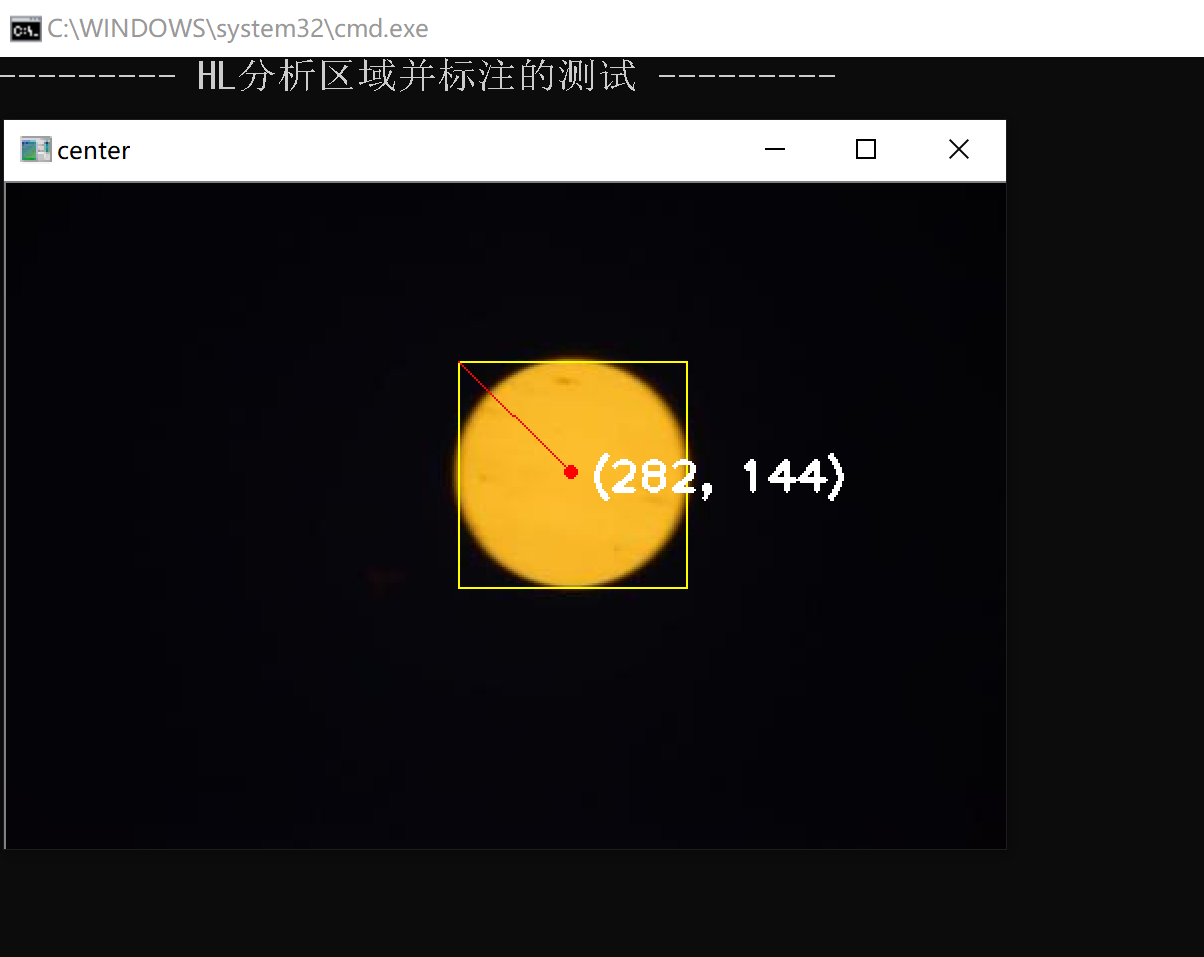# 二、有关代码函数的注释与用法

## 1、cv2.threshold（）阈值处理

``````imgray = cv2.cvtColor(img, cv2.COLOR_BGR2GRAY)#读取并处理为单通道图片，为后续做准备
ret, thresh = cv2.threshold(imgray, 127, 255, 0)#阈值处理
``````

cv2.threshold (src, thresh, maxval, type)

Attention：
src：源图片必须是单通道
thresh：阈值，取值范围0～255
maxval：填充色，取值范围0～255
type：阈值类型，具体见下

0置0置填充色
1置填充色置0
2保持原色置灰色
3置0保持原色
4保持原色置0

``````import cv2

cv2.imshow('org',img)
# 运用二值化处理图片
retVal,img_after = cv2.threshold(img, 100 ,100, cv2.THRESH_BINARY)
cv2.imshow('org_retVal',img_after)

print("变化之前的图像是：\n",img)
print("变化之后的图像是：\n",img_after)

cv2.waitKey(0)
cv2.destroyAllWindows()
``````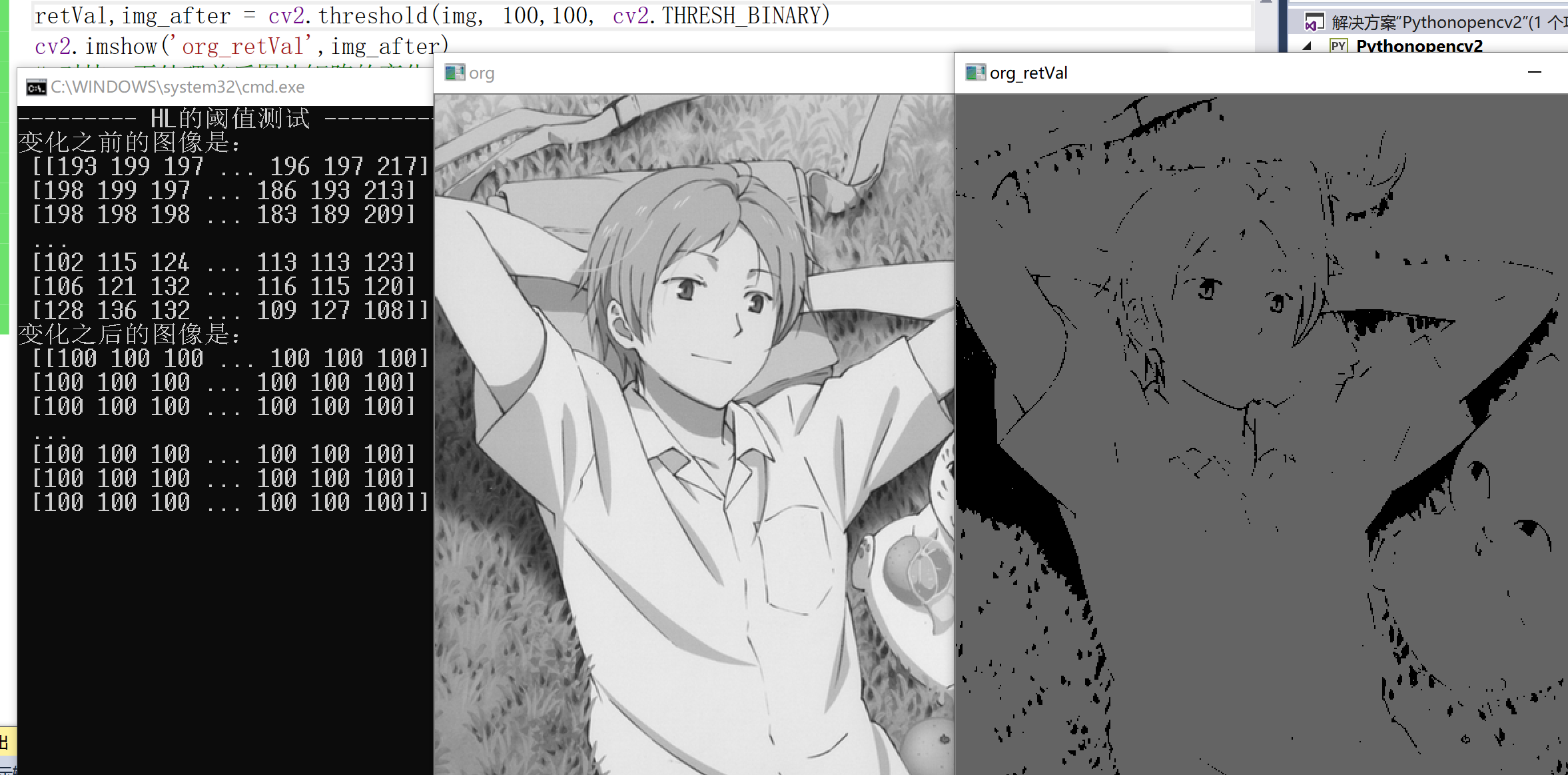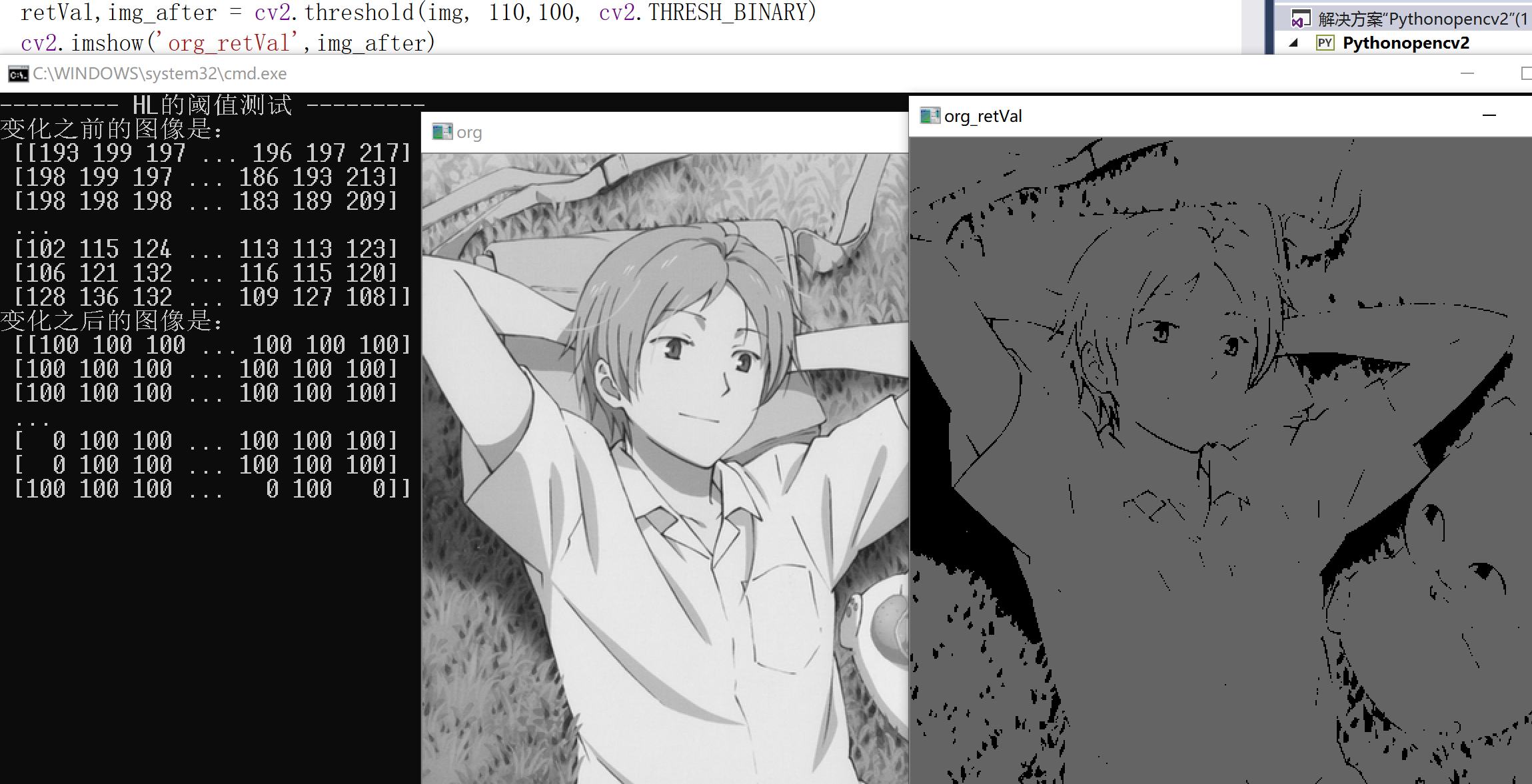## 2、cv2.findContours（）轮廓检测

``````image, contours, hierarchy = cv2.findContours(binary, cv2.RETR_TREE, cv2.CHAIN_APPROX_SIMPLE)
``````

python3这里返回三个值：image,contours,hierarchy

1. image：一张二值图(binary)，但不是灰度图像,应当是黑白图，只有0和1；
2. contours：list结构，列表中每个元素代表一个边沿信息。每个元素是(x,1,2)的三维向量，x表示该条边沿里共有多少个像素点，第三维的那个“2”表示每个点的横、纵坐标；
3. hierarchy：返回类型是(x,4)的二维ndarray。x和contours里的x是一样的意思。如果输入选择cv2.RETR_TREE，则以树形结构组织输出，hierarchy的四列分别对应下一个轮廓编号、上一个轮廓编号、父轮廓编号、子轮廓编号，该值为负数表示没有对应项；

cv2.RETR_EXTERNAL只检测外轮廓
cv2.RETR_LIST只检测外轮廓
cv2.RETR_CCOMP建立两个等级的轮廓，上面的一层为外边界，里面的一层为内孔的边界信息。（如果内孔内还有一个连通物体，这个物体的边界也在顶层）
cv2.RETR_TREE建立一个等级树结构的轮廓

``````import cv2

gray = cv2.cvtColor(img,cv2.COLOR_BGR2GRAY)
ret, binary = cv2.threshold(gray,127,255,cv2.THRESH_BINARY)
cv2.imshow("img", binary)
image,contours, hierarchy = cv2.findContours(binary,cv2.RETR_TREE,cv2.CHAIN_APPROX_SIMPLE)
cv2.drawContours(img,contours,-1,(0,0,225),1)
print("--------- HL边缘检测的测试 ---------")
cv2.imshow("binary", binary)
cv2.waitKey(0)
``````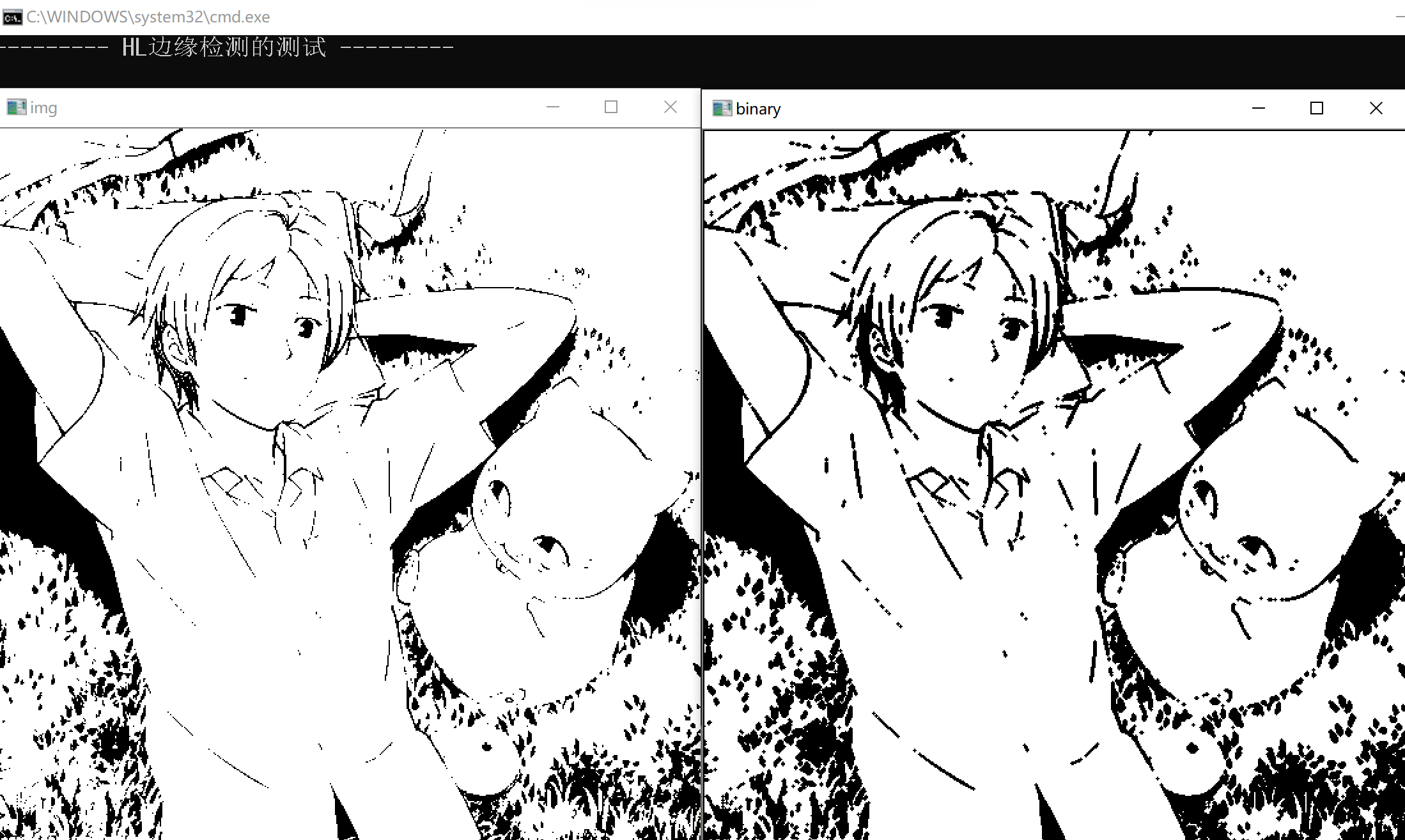``````(x, y, w, h) = cv2.boundingRect(c)
``````

• cnt是一个轮廓点集合，也就是它的参数，可以通过cv2.findContours获取；
• 返回四个值，分别是x，y，w，h；
• x，y是矩阵左上点的坐标；
• w，h是矩阵的宽和高；
``````img = cv2.rectangle(img, (x, y), (x + w, y + h), (0, 255, 255), 1)
``````

shift]]])

• img：要画的圆所在的矩形或图像
• pt1：矩形左上角的点
• pt2：矩形右下角的点
• color：线条颜色，如 (0, 255, 255) 黄色，BGR
• thickness：线条宽度
• lineType：8 (or omitted) ： 8-connected line 4：4-connected line CV_AA - antialiased line
• shift：坐标点小数点位数

## 4、cv2.moments()图像的矩

M = cv2.moments(cnt)

cx = int(M[‘m10’]/M[‘m00’])
cy = int(M[‘m01’]/M[‘m00’])

``````pt = (int(mom['m10'] / mom['m00']), int(mom['m01'] / mom['m00']))  # 使用前三个矩m00, m01和m10计算重心
``````

## 5、cv2.circle（）画圆心

shift]]])

• img：输入的图片data
• center：圆心位置
• color：圆的颜色
• thickness：圆形轮廓的粗细（如果为正）。负厚度表示要绘制实心圆
• lineType： 圆边界的类型。
• shift：中心坐标和半径值中的小数位数。
``````cv2.circle(img, pt, 2, (0, 0, 255), 2)
``````

## 6、 cv2.line（）画直线

`````` cv2.line(img, (x, y),((pt),(pt)), (0, 0, 255),1)
``````

## 7、cv2.putText（）写文字

``````text = "(" + str(pt) + ", " + str(pt) + ")"
cv2.putText(img, text, (pt+10, pt+10), cv2.FONT_HERSHEY_PLAIN, 1.5, (255, 255, 255), 2, 8, 0);
``````

暂无相关的数据...